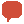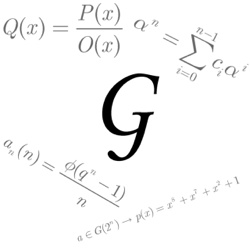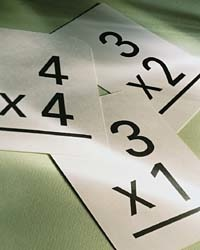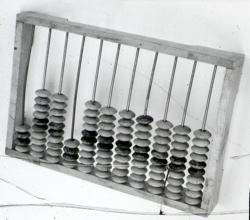Science
Technology |

# 10 Easy Arithmetic TricksListverse Writers. . . Comments

Math can be terrifying for many people. This list will hopefully improve your general knowledge of mathematical tricks and your speed when you need to do math in your head.1. The 11 Times Trick

We all know the trick when multiplying by ten – add 0 to the end of the number, but did you know there is an equally easy trick for multiplying a two digit number by 11? This is it:

Take the original number and imagine a space between the two digits (in this example we will use 52:

5_2

Now add the two numbers together and put them in the middle:

5_(5+2)_2

That is it – you have the answer: 572.

If the numbers in the middle add up to a 2 digit number, just insert the second number and add 1 to the first:

9_(9+9)_9

(9+1)_8_9

10_8_9

1089 – It works every time.

After you understand all the tricks to math, you can proudly wear this Math Ninja T-shirt at Amazon.com!

2. Quick Square

If you need to square a 2 digit number ending in 5, you can do so very easily with this trick. Mulitply the first digit by itself + 1, and put 25 on the end. That is all!

252 = (2x(2+1)) & 25

2 x 3 = 6

625

3. Multiply by 5

Most people memorize the 5 times tables very easily, but when you get in to larger numbers it gets more complex – or does it? This trick is super easy.

Take any number, then divide it by 2 (in other words, halve the number). If the result is whole, add a 0 at the end. If it is not, ignore the remainder and add a 5 at the end. It works everytime:

2682 x 5 = (2682 / 2) & 5 or 0

2682 / 2 = 1341 (whole number so add 0)

13410

Let’s try another:

5887 x 5

2943.5 (fractional number (ignore remainder, add 5)

294354. Multiply by 9

This one is simple – to multiple any number between 1 and 9 by 9 hold both hands in front of your face – drop the finger that corresponds to the number you are multiplying (for example 9×3 – drop your third finger) – count the fingers before the dropped finger (in the case of 9×3 it is 2) then count the numbers after (in this case 7) – the answer is 27.

5. Multiply by 4

This is a very simple trick which may appear obvious to some, but to others it is not. The trick is to simply multiply by two, then multiply by two again:

58 x 4 = (58 x 2) + (58 x 2) = (116) + (116) = 232

Get free shipping, thousands of movies, and millions of streaming songs with a FREE trial of Amazon Prime at Amazon.com!

6. Calculate a Tip

If you need to leave a 15% tip, here is the easy way to do it. Work out 10% (divide the number by 10) – then add that number to half its value and you have your answer:

15% of \$25 = (10% of 25) + ((10% of 25) / 2)

\$2.50 + \$1.25 = \$3.75

7. Tough Multiplication

If you have a large number to multiply and one of the numbers is even, you can easily subdivide to get to the answer:

32 x 125, is the same as:
16 x 250 is the same as:
8 x 500 is the same as:
4 x 1000 = 4,0008. Dividing by 5

Dividing a large number by five is actually very simple. All you do is multiply by 2 and move the decimal point:

195 / 5

Step1: 195 * 2 = 390
Step2: Move the decimal: 39.0 or just 39

2978 / 5

step 1: 2978 * 2 = 5956
Step2: 595.6

9. Subtracting from 1,000

To subtract a large number from 1,000 you can use this basic rule: subtract all but the last number from 9, then subtract the last number from 10:

1000
-648

step1: subtract 6 from 9 = 3
step2: subtract 4 from 9 = 5
step3: subtract 8 from 10 = 2

10. Assorted Multiplication Rules

Multiply by 5: Multiply by 10 and divide by 2.
Multiply by 6: Sometimes multiplying by 3 and then 2 is easy.
Multiply by 9: Multiply by 10 and subtract the original number.
Multiply by 12: Multiply by 10 and add twice the original number.
Multiply by 13: Multiply by 3 and add 10 times original number.
Multiply by 14: Multiply by 7 and then multiply by 2
Multiply by 15: Multiply by 10 and add 5 times the original number, as above.
Multiply by 16: You can double four times, if you want to. Or you can multiply by 8 and then by 2.
Multiply by 17: Multiply by 7 and add 10 times original number.
Multiply by 18: Multiply by 20 and subtract twice the original number (which is obvious from the first step).
Multiply by 19: Multiply by 20 and subtract the original number.
Multiply by 24: Multiply by 8 and then multiply by 3.
Multiply by 27: Multiply by 30 and subtract 3 times the original number (which is obvious from the first step).
Multiply by 45: Multiply by 50 and subtract 5 times the original number (which is obvious from the first step).
Multiply by 90: Multiply by 9 (as above) and put a zero on the right.
Multiply by 98: Multiply by 100 and subtract twice the original number.
Multiply by 99: Multiply by 100 and subtract the original number.

Bonus: Percentages

Yanni in comment 23 gave an excellent tip for working out percentages, so I have taken the liberty of duplicating it here:

Find 7 % of 300. Sound Difficult?

Percents: First of all you need to understand the word “Percent.” The first part is PER , as in 10 tricks per listverse page. PER = FOR EACH. The second part of the word is CENT, as in 100. Like Century = 100 years. 100 CENTS in 1 dollar… etc. Ok… so PERCENT = For Each 100.

So, it follows that 7 PERCENT of 100, is 7. (7 for each hundred, of only 1 hundred).
8 % of 100 = 8. 35.73% of 100 = 35.73
But how is that useful??

Back to the 7% of 300 question. 7% of the first hundred is 7. 7% of 2nd hundred is also 7, and yep, 7% of the 3rd hundred is also 7. So 7+7+7 = 21.

If 8 % of 100 is 8, it follows that 8% of 50 is half of 8 , or 4.

Break down every number that’s asked into questions of 100, if the number is less then 100, then move the decimal point accordingly.

EXAMPLES:
8%200 = ? 8 + 8 = 16.
8%250 = ? 8 + 8 + 4 = 20.
8%25 = 2.0 (Moving the decimal back).
15%300 = 15+15+15 =45.
15%350 = 15+15+15+7.5 = 52.5

Also it’s usefull to know that you can always flip percents, like 3% of 100 is the same as 100% of 3.

35% of 8 is the same as 8% of 35.

Technorati Tags: ,Listverse is a place for explorers. Together we seek out the most fascinating and rare gems of human knowledge. Three awesome top 10 lists daily.